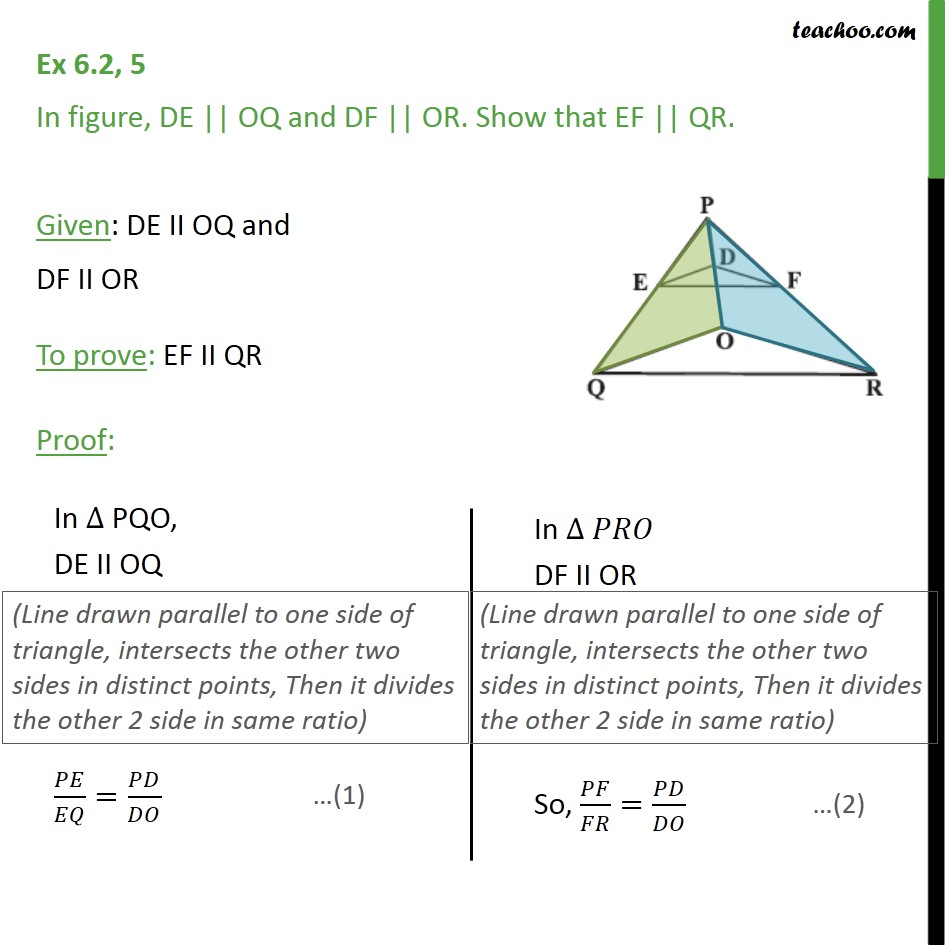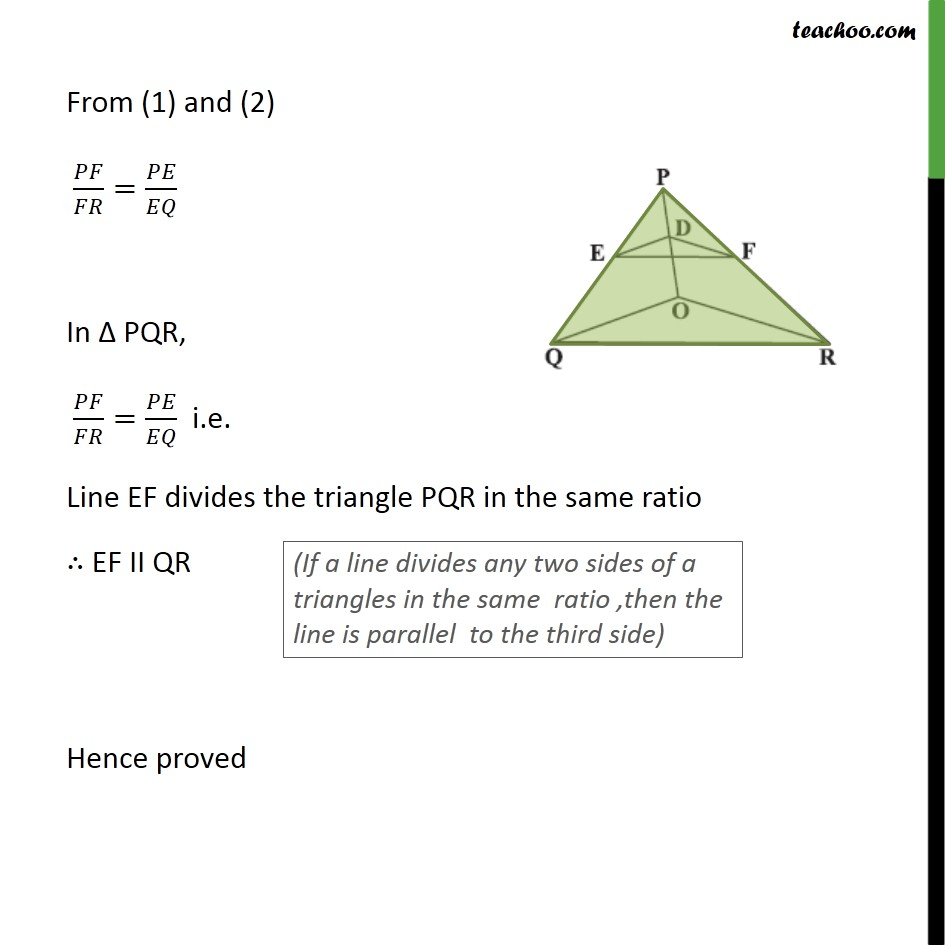1. Chapter 6 Class 10 Triangles (Term 1)
2. Serial order wise
3. Ex 6.2

Transcript

Ex 6.2, 5 In figure, DE || OQ and DF || OR. Show that EF || QR. Given: DE II OQ and DF II OR To prove: EF II QR Proof: From (1) and (2) 𝑃𝐹/𝐹𝑅=𝑃𝐸/𝐸𝑄 In Δ PQR, 𝑃𝐹/𝐹𝑅=𝑃𝐸/𝐸𝑄 i.e. Line EF divides the triangle PQR in the same ratio ∴ EF II QR Hence proved

Ex 6.2